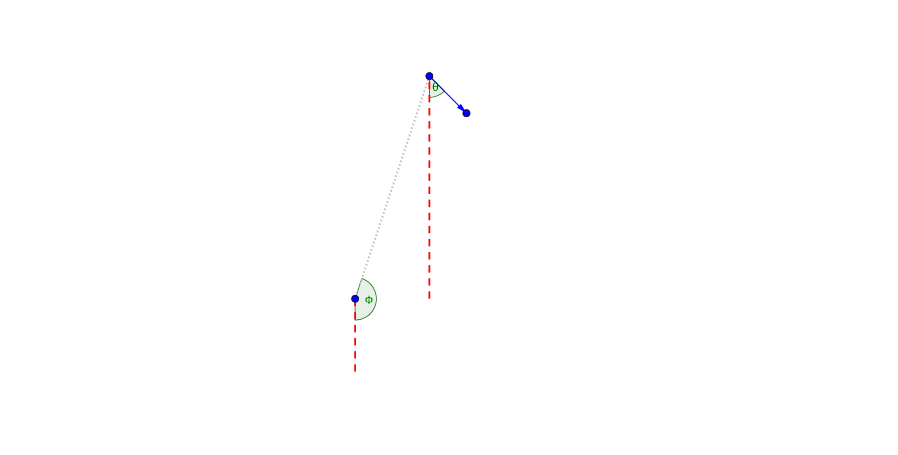# Relativistic Aberration of Light¶Fig. 23 The relativistic aberration of light.

In astrophyics, object moving with a significant fraction of the speed of light $$v_S$$ with angle $$\theta$$ shown in Fig. 23, is measure from the observer in a direction

$\cos \theta_O = \frac{\cos\theta_O - v_S}{1 - v_S \cos \theta_S}.$

If the object is moving towards us, we observe $$\theta_O=0$$.

Meanwhile the apparent transverse velocity is measured to be

(17)$v_{\perp,O} = \frac{v}{1-\beta \cos (\pi - \phi +\theta)},$

where $$\pi - \phi +\theta$$ is the angle between line of sight and the velocity, measured in the object’s frame. One of the astonishing fact about Eq. ((17)) is that it’s maximun value can be larger than 1, which means we could observe superluminal objects.